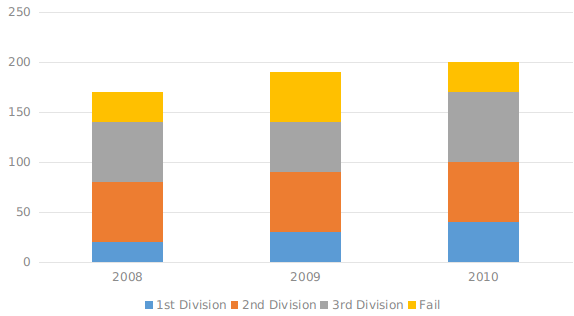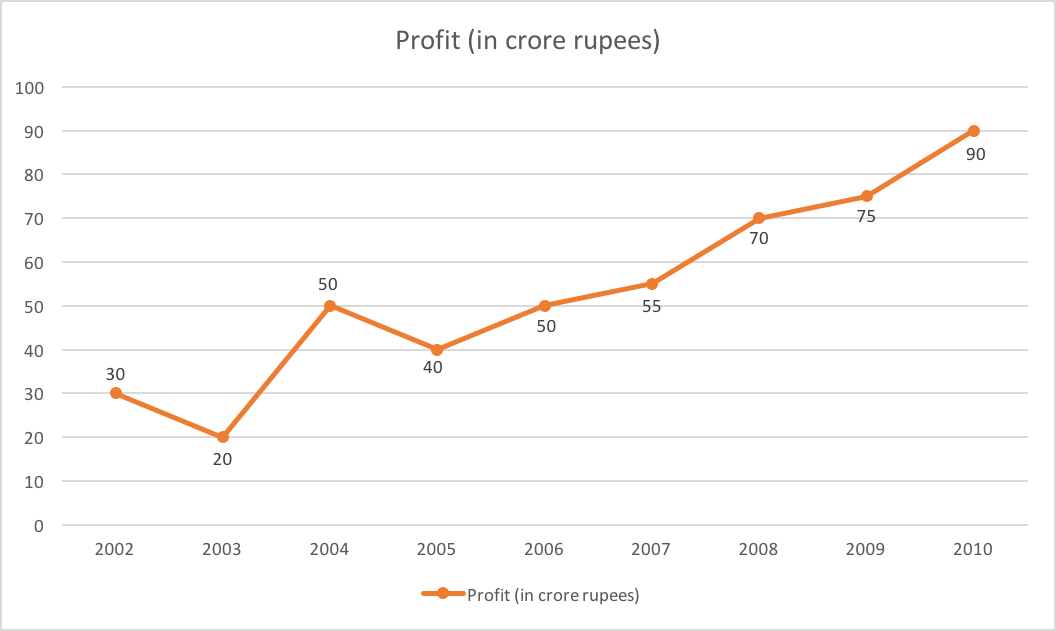0
1455

# Data Interpretation Questions for SSC CHSL set-2 pdf:

For Previous year Data Interpretation Questions of SSC CHSL 2019-2020 Tier I exam download PDF. Go through the video of Repeatedly asked Data Interpretation questions explanations most important for the CHSL exam.

Instructions

The sub divided bar diagram given below depicts H.S. Students of a school for three years. Study the diagram and answer the questions.Question 1: The percentage passed in 1st division in 2008 was

a) 27%

b) 32%

c) $15\frac{3}{8}$ %

d) $11\frac{13}{17}$%

Question 2: The pass percentage in 2008 was

a) 67%

b) 73%

c) $79\frac{2}{3}$%

d) $82\frac{6}{17}$%

Question 3: In which year the school had the best result for H.S. in respect of percentage of pass candidates ?

a) 2008

b) 2009

c) 2010

d) The percentage of pass candidates are same for the three years.

Question 4: The number of students passed in third division in the year 2008 was

a) 50

b) 60

c) 70

d) 80

Question 5: The percentage of the students passed in 2nd division in the year 2010 was

a) 30%

b) 40%

c) 50%

d) 60%

SSC CHSL Study Material (FREE Tests)

Instructions

Study the graph carefully and answer the following questions. Profit Earned by a company (in crore rupees) during certain yearsQuestion 6: If the income in the year 2003 was 60 crores and the expenditure of that year equals the expenditure of the year 2005, the income of the company (in crore rupees) in 2005 was

a) 75

b) 80

c) 65

d) 70

Question 7: If the expenditure in the year 2007 was 60 crores, then the income (in crore rupees) in that year was

a) 115

b) 120

c) 85

d) 90

Question 8: The year in which the percentage increase in the profit from that of the previous year is the highest is

a) 2006

b) 2008

c) 2002

d) 2004

Question 9: What is the approximate per cent (to the nearest integer) r,r it of the year 2006, if the inc. —ne of that year was? 120 crores ?

a) 42%

b) 51%

c) 41%

d) 43%

Question 10: What is the approximate per cent (to nearest integer) increase in the profit in the year
2009 from that of the previous year ?

a) 9%

b) 10%

c) 7%

d) 8%

no.of students passed in 1st division in 2008 = 20

total no.of students in 2008 = 170

The percentage passed in 1st division in 2008 = $\frac{20}{170}X100$ % = $11\frac{13}{17}$ %

so the answer is option D.

students passed in 2008 = 140 out of 170

pass percentage = $\frac{140}{170}\times100=82\frac{6}{17}$%

so the answer is option D.

pass percentage = $\frac{no.of passed candidates}{total no.of candidates}\times100$

pass percentage in 2008 = $\frac{140}{170}\times100=82.35$%

pass percentage in 2009 = $\frac{140}{190}\times100=73.68$%

pass percentage in 2010 = $\frac{170}{200}\times100=85$%

so the answer is option A.

from the graph,

The number of students passed in third division in the year 2008 was 140 – 80 = 60

so the answer is option B.

students passed in 2nd division in the year 2010 = 60

students appeared for exam  in the year 2010 = 200

percentage = $\frac{60}{200}\times100=30$%

so the answer is option A.

profit in 2003 = 20cr

if income in 2003 = 60cr, then expenditure = 60 – 20 = 40cr

according to question, expenditure in 2005 = expenditure in 2002 = 40crs

from graph, profit in 2005 = 40cr

so income in 2005 = profit + expenditure = 40+40 = 80cr

so the answer is option B.

profit = income-expenditure

55 = Income-60

Income = 115

so the answer is option A.

profit increased only in the years 2004, 2006, 2007, 2008, 2009 & 2010.

percentage increase in the profit in 2004 = $\frac{50-20}{20}\times100=\frac{30}{20}\times100=150$%

percentage increase in the profit in 2006 = $\frac{50-40}{40}\times100=\frac{10}{40}\times100=25$%

percentage increase in the profit in 2007 = $\frac{55-50}{50}\times100=\frac{5}{50}\times100=10$%

percentage increase in the profit in 2008 = $\frac{70-55}{55}\times100=\frac{15}{55}\times100=27.27$%

percentage increase in the profit in 2009 = $\frac{75-70}{70}\times100=\frac{5}{70}\times100=7.14$%

percentage increase in the profit in 2010 = $\frac{90-75}{75}\times100=\frac{15}{75}\times100=20$%

so the answer is option D.

% increase in profit = $\frac{5}{70}\times100=7.142\sim7$